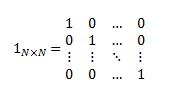## Excel Office

Excel How Tos, Tutorials, Tips & Tricks, Shortcuts

# MUNIT function: Description, Usage, Syntax, Examples and Explanation

## What is MUNIT function in Excel?

MUNIT function is one of the Math and Trig functions in Microsoft Excel that returns the unit matrix for the specified dimension.

## Syntax of MUNIT function

MUNIT(dimension)

The MUNIT function syntax has the following arguments.

Dimension: Dimension is an integer specifying the dimension of the unit matrix that you want to return. It returns an array. The dimension has to be greater than zero.

MUNIT uses the following equation:## MUNIT formula explanation

• If dimension is a value that’s equal to or smaller than zero (0), MUNIT returns the #VALUE! error value.

## Example of MUNIT function

Steps to follow:

1. Open a new Excel worksheet.

2. Copy data in the following table below and paste it in cell A1

Note: For formulas to show results, select them, press F2 key on your keyboard and then press Enter.

You can adjust the column widths to see all the data, if need be.

 Formula Description Results are the 3×3 matrix below, in cells C2:E4. ‘=MUNIT(3) Returns the identity matrix of dimension 3. =MUNIT(3) =MUNIT(3) =MUNIT(3) =MUNIT(3) =MUNIT(3) =MUNIT(3) =MUNIT(3) =MUNIT(3) =MUNIT(3) ‘=MMULT({1,3;5,12}, MUNIT(2))={1,3;5,12} MUNIT can be used in line with other matrix functions, such as MMULT, as shown here. =MMULT({1,3;5,12}, MUNIT(2))={1,3;5,12}

Note: To return a 3×3 matrix, the formula in C2:E4 needs to be entered as an array by pressing Ctrl+Shift+Enter.